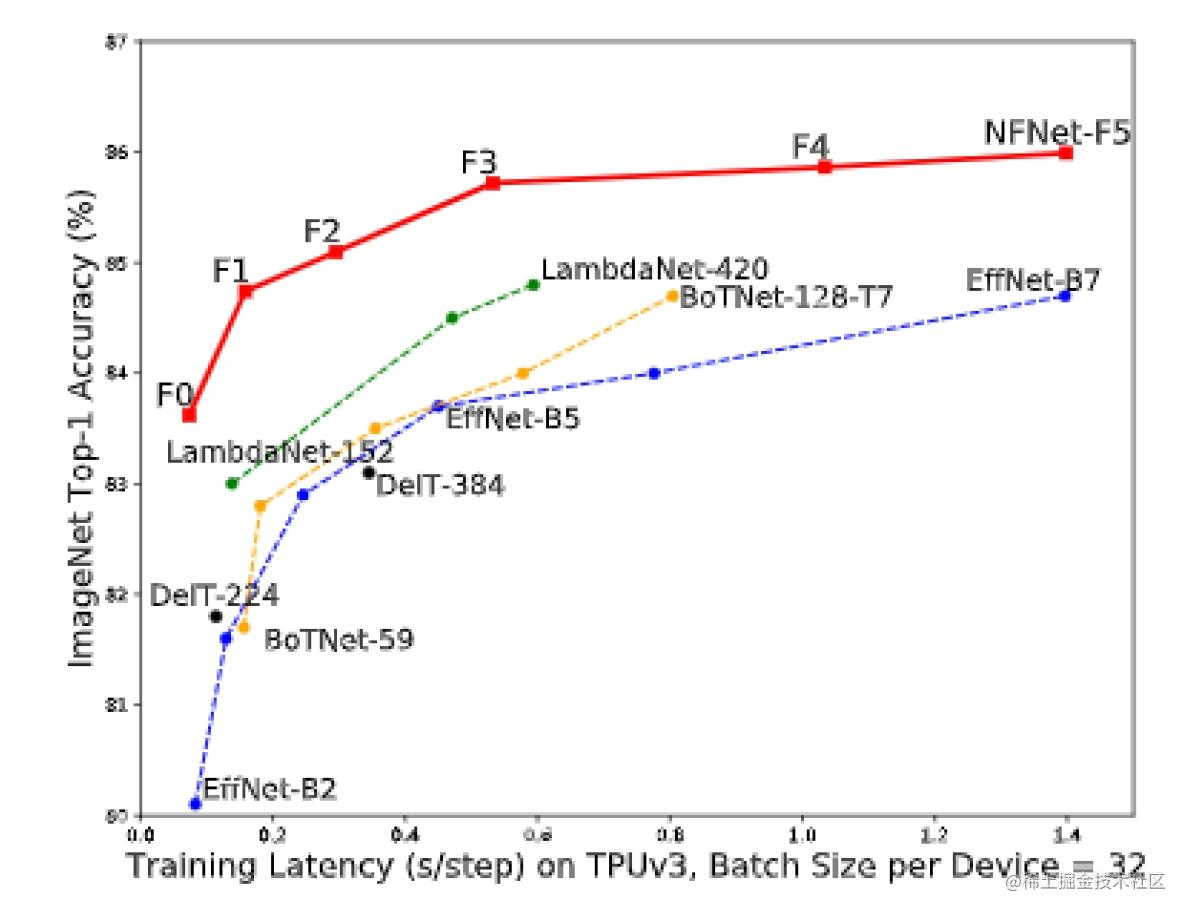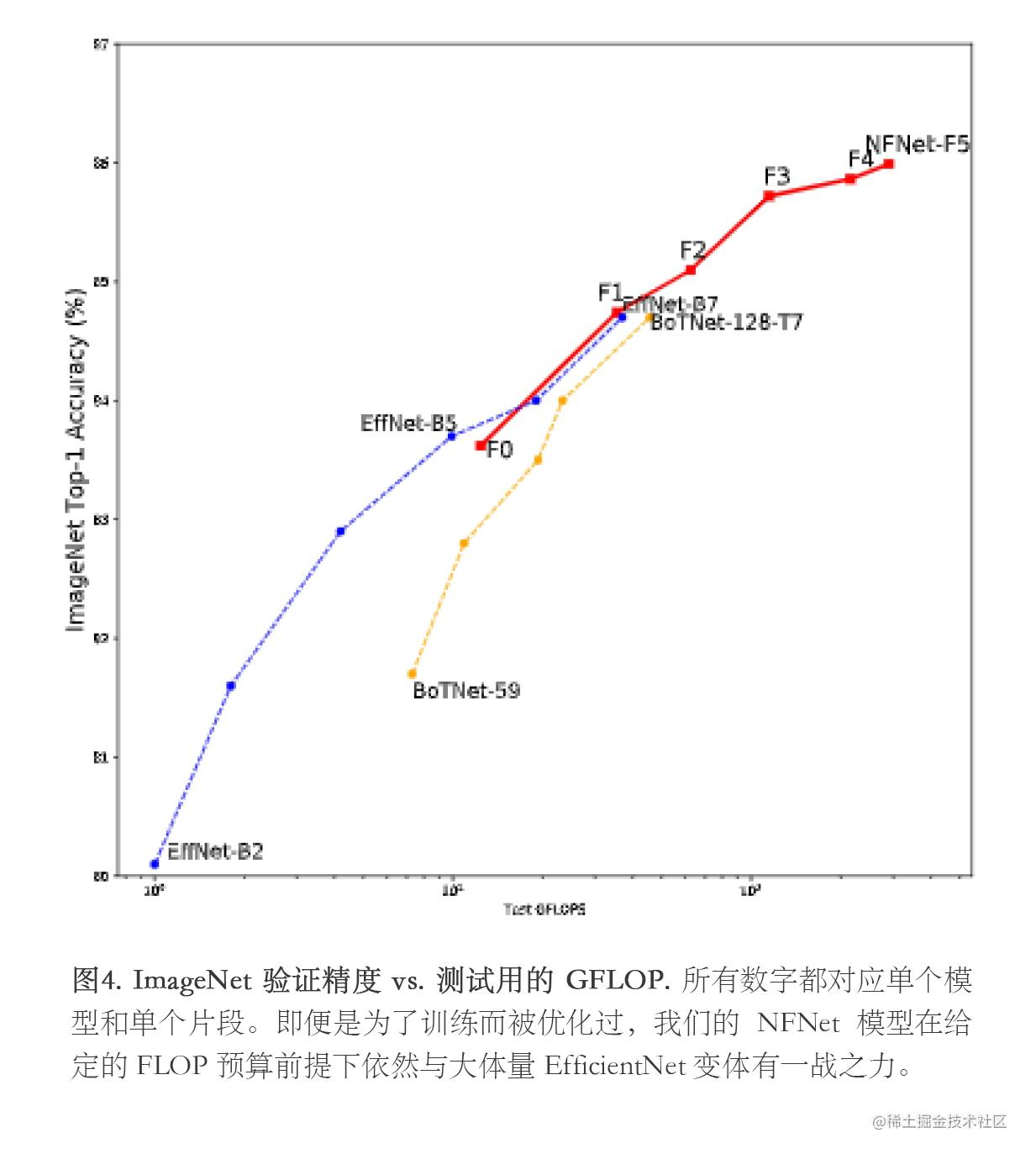# 谷歌 DeepMind 发布 NFNet：高效的深度网络

NFNet-F1 模型达到了和 EfficientNet-B7 相近的精度，但训练速度却比后者快了 8.7 倍。而且我们最大的模型在不利用额外数据的情况下 top-1 精度达到了 86.5%，树立了新的前沿标准。DeepMind 提出了 NFNet 以将标准化从等式中移除并提高训练表现。此外，该公司还提出了一项技术名为``````def forward(inputs, is_training):
model = nfnet.NFNet(num_classes=1000,  variant=variant)
return model(inputs, is_training=is_training)['logits']
net = hk.without_apply_rng(hk.transform(forward))
fwd = jax.jit(lambda inputs: net.apply(params, inputs, is_training=False))
# 我们将它分成两个 cell 以便我们不用重复地对 fwd fn 进行 jit 操作。
logits = fwd(x[None]) # 给 X 一个新的维度来让它的 batch size 变成 1。
which_class = imagenet_classlist[int(logits.argmax())]
print(f'ImageNet class: {which_class}.')

NFNet 也有一个 Pytorch 实现版本，这表明社区已经接纳了这个发行版：

``````import torch
from torch import nn, optim
from torchvision.models import resnet18

from nfnets import WSConv2d
from nfnets.agc import AGC # 需要测试

conv = nn.Conv2d(3,6,3)
w_conv = WSConv2d(3,6,3)

optim = optim.SGD(conv.parameters(), 1e-3)
optim_agc = AGC(conv.parameters(), optim) # 需要测试

# 应用 AGC 时，忽略模型的 fc。
model = resnet18()
optim = torch.optim.SGD(model.parameters(), 1e-3)
optim = AGC(model.parameters(), optim, model=model, ignore_agc=['fc'])